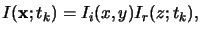Next: 5.2.2 Scaled Defocus Method Up: 5.2 Field Calculation over Previous: 5.2 Field Calculation over

## 5.2.1 Vertical Propagation Method

The vertical propagation method is the simplest imaging model. It is derived from the thin-film theory of Paul Berning  and was introduced into lithography simulation by Frederick Dill . Early versions of the popular lithography simulators PROLITH  and SAMPLE  were based on it. The latent bulk image is obtained by the separation approach(5.24)

whereby Ii(x, y) denotes the aerial image intensity and Ir(z;tk) is the standing wave intensity inside the resist at a certain time-step tk. For the computation of Ir(z;tk) the resist is divided into thin layers over which the intensity and thus also the refractive index n(z;tk) is approximately constant. Within one layer a technique similar to that described in Appendix C is employed to compute the field intensity. However, the method implicitly assumes that the field propagates only in vertical direction. For very low numerical apertures and reasonably thin resists this assumption is valid. It starts to fail when the aerial image changes or defocuses'' as it penetrates into the resist or when the incident light is appreciably oblique.Next: 5.2.2 Scaled Defocus Method Up: 5.2 Field Calculation over Previous: 5.2 Field Calculation over
Heinrich Kirchauer, Institute for Microelectronics, TU Vienna
1998-04-17